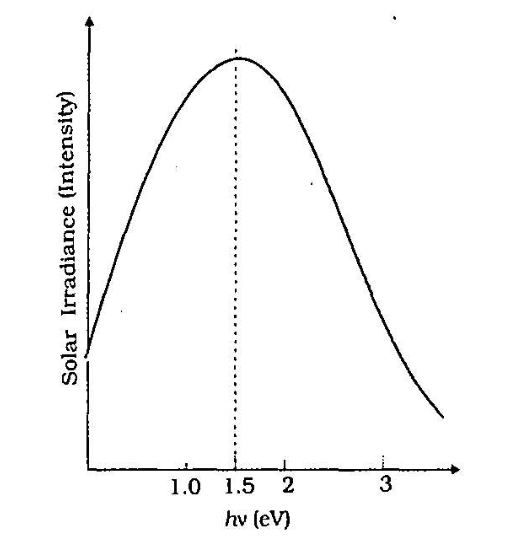Home/Class 12/Physics/

## QuestionPhysicsClass 12

Why are $$\mathit{Si}$$ and $$\mathit{GaAs}$$  are preferred materials for solar cells?The maxima is near $$1.5\mathit{eV}$$ . For photo-excitation. $$\mathit{hv}>E_g$$ . Hence, semiconductor with band gap $${\sim}1.5$$   $$\mathit{eV}$$  or lower is likely to give better solar conversion effleiency. Sillicon has $$E_g{\sim}1.1$$   $$\mathit{eV}$$  while for $$\mathit{GaAs}$$  it is $$-1.53\mathit{eV.}$$  In fact, $$\mathit{GaAs}$$  is better $$($$ in spite of its higher band gap $$)$$ than $$\mathit{Si}$$  because of its relatively higher absorption coefficient. If we choose materials like CdS or CdSe $$\left(E_g-2.4\mathit{eV}\right)$$ . we can use only the high energy component of the solar energy for photo-conversion and a signiflcant part of energy will be of no use. The question arises: why we do not use material like $$\mathit{PbS}\left(E_g{\sim}0.4\mathit{eV}\right)$$  which satisfy the condition $$\mathit{hv}>E_g$$  for $$v$$  maxima corresponding to the solar radiation spectra? If we do so. most of the solar radiation will be absorbed on the top-layer of solar cell and will not reach in or near the depletion region. For effective electron-hole separation. due to the junction field. we want the photo-generation to occur in the junction region only.